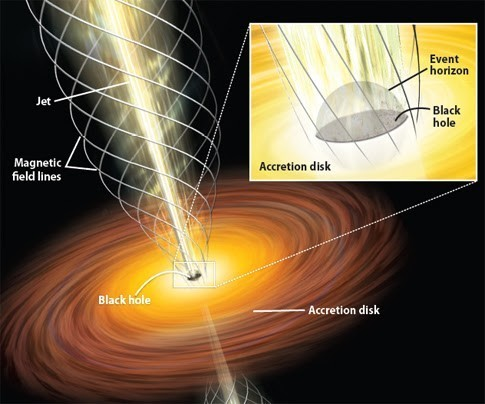# Point of No Return

In Black Holes by Brian Koberlein1 Comment

If you toss a ball up into the air, it will fall back to the ground. Toss one faster, and the ball will travel higher before returning to the ground. Of course this raises the question of just how fast you would have to throw a ball for it to never fall back down. Put another way, could you throw a ball so fast that it would escape the Earth’s gravitational pull forever?

In practice the answer to this question is fairly complicated, because things like air resistance would slow the ball down, and calculating the air resistance of a ball depends on its speed, the density of air, etc. But we can calculate the speed for the hypothetical case where we only need to overcome gravity. In that case the answer is really simple. It is just the square root of the planet’s surface gravity times its diameter.

In the case of the Earth, the surface gravity is about 9.8 m/s, and its diameter is about 12.7 million meters, so if we multiply these numbers together and take the square root, we get a speed of about 11 km/s (or about 25,000 mph). This is known as the escape velocity. At that speed, the ball would have enough energy to overcome the Earth’s gravitational pull.

Since the surface gravity depends on mass and size, you can actually calculate the escape velocity of a (spherical, non-rotating) planet or star simply by knowing its mass and radius. The escape velocity increases for a larger mass or a smaller radius. For example, the escape velocity of the Sun is about 620 km/s, but a white dwarf with the same mass has an escape velocity of about 6,000 km/s, since it is about a hundredth the size of the Sun.

If a mass like the Sun were squeezed smaller and smaller, the escape velocity would get faster and faster. Of course there is a limit to how fast you can go, which is the speed of light. If an object had an escape velocity faster than light, then nothing could escape its gravitational pull. If you think such an object would be a black hole, you’re almost right.

In our hypothetical example, if the escape velocity were the speed of light, we could still toss an object in the air. If we tossed a ball at a speed approaching the speed of light, it would travel very far from the surface before falling back to the ground. Relativistic gravity doesn’t work that way, but our simple model actually gives us clues about relativistic gravity.

For any given mass, we can calculate the size we would have to squeeze it down to for the escape velocity to be the speed of light. For the Sun, we’d need to squeeze it into a sphere with a radius of about 3 kilometers. This radius is known as the Schwarzschild radius of the Sun.

For every mass you can calculate a Schwarzschild radius. This means that the escape velocity can be used to relate the mass of an object to a distance. In other words, for every mass there is a corresponding length. General relativity describes gravity as a warping of space, and this connection between mass and Schwarzschild radius shows up again.

In general relativity, the radius of a (non-rotating) black hole is the Schwarzschild radius of its mass. It’s tempting to say that the escape velocity of a black hole is the speed of light, but that’s not quite how it works. For a black hole, the Schwarzschild radius defines the surface known as the event horizon. If you were to cross the event horizon of a black hole, you would be forever trapped. Space is warped in such a way you can only move inward, never outward. Once in a black hole you are trapped in an ever shrinking sphere.

Of course this also means that anything crossing the event horizon of a black hole is unable to provide any information to us (at least in the classical sense). We could never see past a black hole’s event horizon, so we can’t be sure what lies inside. This is why the “size” of a black hole is often given by its event horizon. It is not a physical surface, but a surface beyond which we have no information.

It is the point of no return.

1.